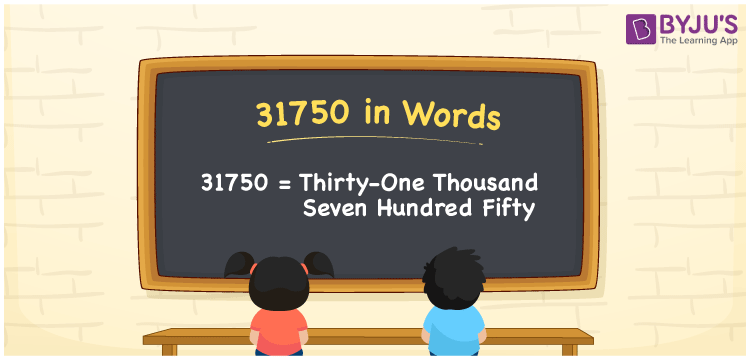# 31750 in Words

The number name of 31750 is Thirty-one thousand seven hundred fifty. As we know, the number 31750 is a cardinal number and its place value helps to write the number name. For example, there are 31750 books in a library. In this article, let us discuss the process of writing the number 31750 in words using the place value system.

 31750 in Words: Thirty-one Thousand Seven Hundred Fifty. Thirty-one Thousand Seven Hundred Fifty in Numerical Form: 31750.

## 31750 in English Words## How to Write 31750 in Words?

Below table is the place value table for the number 31750:

 Ten-thousands Thousands Hundreds Tens Ones 3 1 7 5 0

The expanded form of 31750 is as follows:

= 3 × Ten thousand + 1 × Thousand + 7 × Hundred + 5 × Ten + 0 × One

= 3 × 10000 + 1 × 1000 + 7 × 100 + 5 × 10 + 0 × 1

= 30000 + 1000 + 700 + 50

= 31750

= Thirty-one thousand seven hundred fifty

Hence, 31750 in words is Thirty-one thousand seven hundred fifty.

31750 in words – Thirty-one thousand seven hundred fifty

Is 31750 an odd number? – No

Is 31750 an even number? – Yes

Is 31750 a perfect square number? – No

Is 31750 a perfect cube number? – No

Is 31750 a prime number? – No

Is 31750 a composite number? – Yes

## Frequently Asked Questions on 31750 in Words

Q1

### Write 31750 in words.

31750 in words is thirty-one thousand seven hundred fifty.

Q2

### Simplify 31000 + 750, and express it in words.

Simplifying 31000 + 750, we get 31750. Hence, 31750 in words is thirty-one thousand seven hundred fifty.

Q3

### Is 31750 an odd number?

No, 31750 is not an odd number.